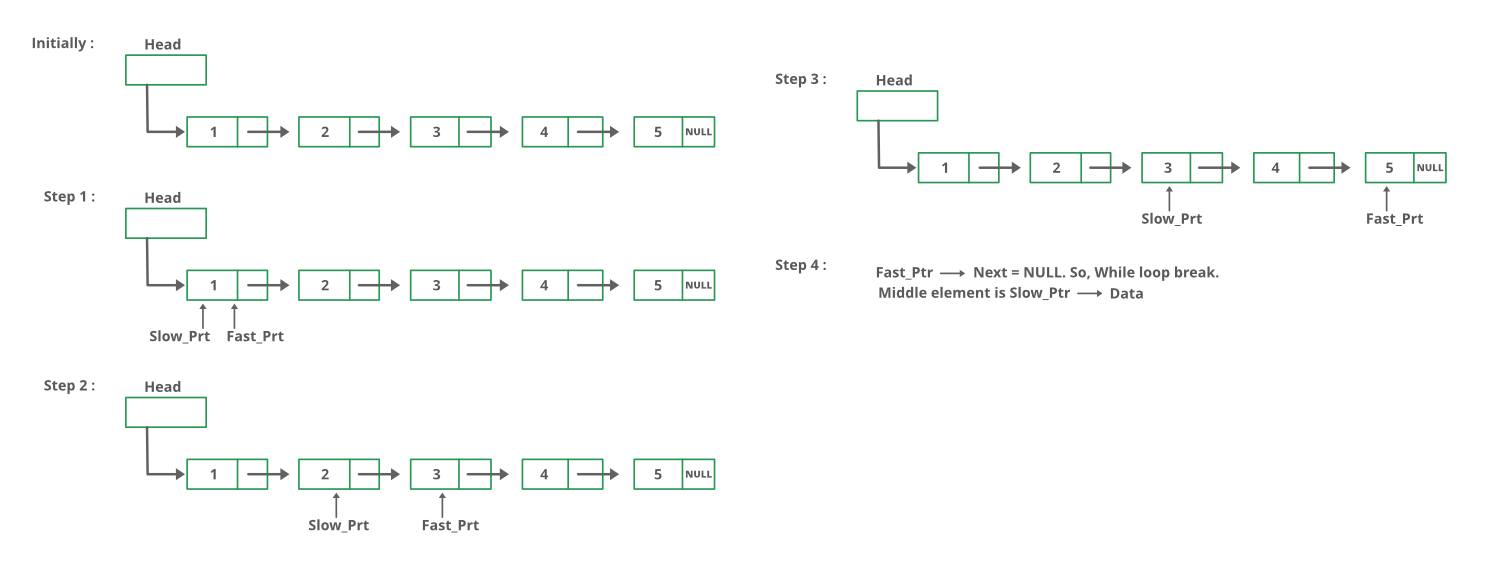GeeksforGeeks App
Open AppBrowser
Continue

# Python Program For Finding The Middle Element Of A Given Linked List

Given a singly linked list, find the middle of the linked list. For example, if the given linked list is 1->2->3->4->5 then the output should be 3.
If there are even nodes, then there would be two middle nodes, we need to print the second middle element. For example, if given linked list is 1->2->3->4->5->6 then the output should be 4.

Method 1:
Traverse the whole linked list and count the no. of nodes. Now traverse the list again till count/2 and return the node at count/2.

Method 2:
Traverse linked list using two pointers. Move one pointer by one and the other pointers by two. When the fast pointer reaches the end slow pointer will reach the middle of the linked list.

Below image shows how printMiddle function works in the code :## Python3

 `# Python3 program to find middle of``# the linked list``# Node class``class` `Node:``  ` `    ``# Function to initialise the``    ``# node object``    ``def` `__init__(``self``, data):``   ` `        ``# Assign data``        ``self``.data ``=` `data` `        ``# Initialize next as null ``        ``self``.``next` `=` `None` `  ` `# Linked List class contains a``# Node object``class` `LinkedList:``  ` `    ``# Function to initialize head``    ``def` `__init__(``self``):``        ``self``.head ``=` `None` `    ``# Function to insert a new node at``    ``# the beginning ``    ``def` `push(``self``, new_data): ``        ``new_node ``=` `Node(new_data) ``        ``new_node.``next` `=` `self``.head ``        ``self``.head ``=` `new_node` `    ``# Print the linked list``    ``def` `printList(``self``):``        ``node ``=` `self``.head``        ``while` `node:``            ``print``(``str``(node.data) ``+``                  ``"->"``, end ``=` `"")``            ``node ``=` `node.``next``        ``print``(``"NULL"``)` `    ``# Function that returns middle.``    ``def` `printMiddle(``self``):` `        ``# Initialize two pointers, one will go``        ``# one step a time (slow), another two``        ``# at a time (fast)``        ``slow ``=` `self``.head``        ``fast ``=` `self``.head` `        ``# Iterate till fast's next is null (fast``        ``# reaches end)``        ``while` `fast ``and` `fast.``next``:``            ``slow ``=` `slow.``next``            ``fast ``=` `fast.``next``.``next``        ` `        ``# Return the slow's data, which would be``        ``# the middle element.``        ``print``(``"The middle element is "``, slow.data)` `# Driver code``if` `__name__``=``=``'__main__'``:``  ` `    ``# Start with the empty list``    ``llist ``=` `LinkedList()``  ` `    ``for` `i ``in` `range``(``5``, ``0``, ``-``1``):``        ``llist.push(i)``        ``llist.printList()``        ``llist.printMiddle()` `# This code is contributed by Kumar Shivam (kshivi99)`

Output:

```5->NULL
The middle element is 

4->5->NULL
The middle element is 

3->4->5->NULL
The middle element is 

2->3->4->5->NULL
The middle element is 

1->2->3->4->5->NULL
The middle element is ```

Time Complexity: O(n) where n is the number of nodes in the given linked list.
Auxiliary Space: O(1), no extra space is required, so it is a constant.

Method 3:
Initialize mid element as head and initialize a counter as 0. Traverse the list from head, while traversing increment the counter and change mid to mid->next whenever the counter is odd. So the mid will move only half of the total length of the list.
Thanks to Narendra Kangralkar for suggesting this method.

## Python3

 `# Python program to implement``# the above approach` `# Node class``class` `Node:``   ` `    ``# Function to initialise the``    ``# node object``    ``def` `__init__(``self``, data):` `        ``# Assign data ``        ``self``.data ``=` `data ` `        ``# Initialize next as null``        ``self``.``next` `=` `None` `   ` `# Linked List class contains a``# Node object``class` `LinkedList:``   ` `    ``# Function to initialize head``    ``def` `__init__(``self``):``        ``self``.head ``=` `None`` ` `    ``# Function to insert a new node at``    ``# the beginning ``    ``def` `push(``self``, new_data): ``        ``new_node ``=` `Node(new_data) ``        ``new_node.``next` `=` `self``.head ``        ``self``.head ``=` `new_node`` ` `    ``# Print the linked list``    ``def` `printList(``self``):``        ``node ``=` `self``.head``        ``while` `node:``            ``print``(``str``(node.data) ``+``                      ``"->"``, end ``=` `"")``            ``node ``=` `node.``next``        ``print``(``"NULL"``)`` ` `    ``# Function to get the middle of``    ``#  the linked list``    ``def` `printMiddle(``self``):``        ``count ``=` `0``        ``mid ``=` `self``.head``        ``heads ``=` `self``.head``  ` `        ``while``(heads !``=` `None``):``      ` `        ``# Update mid, when 'count'``        ``# is odd number``            ``if` `count & ``1``:``                ``mid ``=` `mid.``next``            ``count ``+``=` `1``            ``heads ``=` `heads.``next``            ` `        ``# If empty list is provided``        ``if` `mid !``=` `None``:``            ``print``(``"The middle element is "``,``                   ``mid.data)`` ` `# Driver code``if` `__name__``=``=``'__main__'``:``   ` `    ``# Start with the empty list``    ``llist ``=` `LinkedList()``   ` `    ``for` `i ``in` `range``(``5``, ``0``, ``-``1``):``        ``llist.push(i)``        ``llist.printList()``        ``llist.printMiddle()`` ` ` ``# This code is contributed by Manisha_Ediga`

Output:

```5->NULL
The middle element is 

4->5->NULL
The middle element is 

3->4->5->NULL
The middle element is 

2->3->4->5->NULL
The middle element is 

1->2->3->4->5->NULL
The middle element is ```

Time Complexity: O(n) where n is the number of nodes in the given linked list.
Auxiliary Space: O(1), no extra space is required, so it is a constant.

Please refer complete article on Find the middle of a given linked list for more details!

My Personal Notes arrow_drop_up